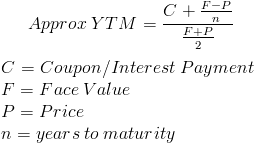Skip to content

# Yield to Maturity

Yield to maturity (YTM) is the total return anticipated on a bond if the bond is held until it matures. Yield to maturity is considered a long-term bond yield but it is expressed as an annual rate. In other words, it is the internal rate of return (IRR) of an investment in a bond if the investor holds the bond until maturity, with all payments made as scheduled and reinvested at the same rate.

Because yield to maturity is the interest rate an investor would earn by reinvesting every coupon payment from the bond at a constant interest rate until the bond’s maturity date, the present value of all the future cash flows equals the bond’s market price. An investor knows the current bond price, its coupon payments and its maturity value, but the discount rate cannot be calculated directly. However, there is a trial-and-error method for finding YTM with the following present value formula:Alternative formula for finding YTM

Each one of the future cash flows of the bond is known and because the bond’s current price is also known, a trial-and-error process can be applied to the YTM variable in the equation until the present value of the stream of payments equals the bond’s price.

Solving the equation by hand requires an understanding of the relationship between a bond’s price and its yield, as well as of the different types of bond pricings. Bonds can be priced at a discount, at par or at a premium. When the bond is priced at par, the bond’s interest rate is equal to its coupon rate. A bond priced above par, called a premium bond, has a coupon rate higher than the realized interest rate and a bond priced below par, called a discount bond, has a coupon rate lower than the realized interest rate. If an investor were calculating YTM on a bond priced below par, he or she would solve the equation by plugging in various annual interest rates that were higher than the coupon rate until finding a bond price close to the price of the bond in question.

Calculations of yield to maturity (YTM) assume that all coupon payments are reinvested at the same rate as the bond’s current yield and take into account the bond’s current market price, par value, coupon interest rate and term to maturity. The YTM is merely a snapshot of the return on a bond because coupon payments cannot always be reinvested at the same interest rate. As interest rates rise, the YTM will increase; as interest rates fall, the YTM will decrease.

### 2 Comments »

error: Content is protected !!